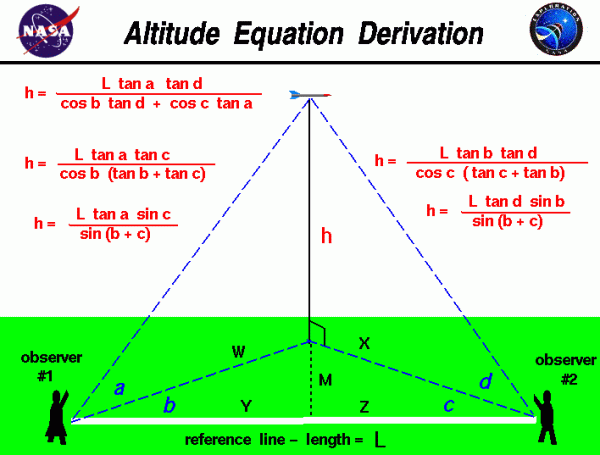# Altitude Equation Derivation## Flying Model Rocket

Flying model rockets is a relatively safe and inexpensive way for students to learn the basics of forces and the response of vehicles to external forces. Students can also use math techniques learned in high school to determine the performance of the rocket during the flight.

## Determining Maximum Altitude

On this page we derive the equations which are shown on the figure to determine the maximum altitude the rocket reaches during the flight. The procedure requires two observers to measure several angles. The observers are placed some distance L apart along a reference line which is shown in white on the figure. As the rocket passes its maximum altitude, observer #1 measures the angle a between the ground and the rocket. This measurement is taken perpendicular to the ground. Observer #1 then measures the angle b between the rocket and the reference line. This measurement is taken parallel to the ground. The second observer measures the angle d from the ground to the rocket and the angle c, parallel to the ground, between the direction the observer is facing and the reference line.

## Deriving the Equations

With the four measured angles and the measured distance between the observers, we can use some relations from trigonometry to derive the equation for the altitude h. We will need four “construction” triangles to derive the equation. The first two triangles are formed by the altitude h, the line of sight from the observers to the rocket, and the ground track of the line of sight.

From observer #1, we have our first trigonometry relations:

#### Eq. 1:

$$\LARGE \frac{h}{w}=\tan a$$

#### Eq. 1a:

$$\LARGE h=w\tan a$$

#### Eq. 1b:

$$\LARGE w=\frac{h}{\tan a}$$

From observer #2:

#### Eq. 2:

$$\LARGE \frac{h}{x}=\tan d$$

#### Eq. 2a:

$$\LARGE h=x\tan d$$

#### Eq. 2b:

$$\LARGE x=\frac{h}{\tan d}$$

If we drop a perpendicular line from the base of the altitude to the reference line, we construct two triangles with the following properties:

#### Eq. 3:

$$\LARGE \frac{y}{w}=\cos b$$

#### Eq. 3a:

$$\LARGE y=w\cos b$$

#### Eq. 4:

$$\LARGE \frac{m}{w}=\sin b$$

#### Eq. 4a:

$$\LARGE m=w\sin b$$

#### Eq. 5:

$$\LARGE \frac{z}{x}=\cos c$$

#### Eq. 5a:

$$\LARGE z=x\cos c$$

#### Eq. 6:

$$\LARGE \frac{m}{x}=\sin c$$

#### Eq. 6a:

$$\LARGE m=x\sin c$$

#### Eq. 7:

$$\LARGE L=y+z$$

We will start with Eq. 7 and substitute the value of y from Eq, 3a and the value of z from Eq, 5a:

#### Eq. 8:

$$\LARGE L=w\cos b+x\cos c$$

Now substitute the value for w from Eq. 1b and the value of x from Eq. 2b:

#### Eq. 9:

$$\LARGE L=h\frac{\cos b}{\tan a}+h\frac{\cos c}{\tan d}$$

#### Eq. 9a:

$$\LARGE L=h(\frac{\cos b}{\tan a}+\frac{\cos c}{\tan d})$$

Solve for h:

#### Eq. 10:

$$\LARGE h=\frac{L\tan a\tan d}{\cos b\tan d+\cos c\tan a}$$

A detailed analysis of this trigonometry problem indicates that we really only need three angle measurements along with the reference length measurement to completely determine the answer. The angles a, bc, and d are related to each other and we can eliminate one of the angle measurements and still determine the altitude.

We can equate the values of h from Eq. 1a and Eq. 2a:

#### Eq. 11:

$$\LARGE h=w\tan a=x\tan d$$

#### Eq. 11a:

$$\LARGE \frac{w}{x}=\frac{\tan d}{\tan a}$$

We can derive another relationship for w/x by using Eq. 4a and Eq. 6a:

#### Eq. 12:

$$\LARGE m=w\sin b=x\sin c$$

#### Eq. 12a:

$$\LARGE \frac{w}{x}=\frac{\sin c}{\sin b}$$

Then:

#### Eq. 13:

$$\LARGE \frac{\tan d}{\tan a}=\frac{\sin c}{\sin b}$$

#### Eq. 13a:

$$\LARGE \tan d=\frac{\sin c}{\sin b}\tan a$$

#### Eq. 13b:

$$\LARGE \tan a=\frac{\sin b}{\sin c}\tan d$$

We can eliminate angle a from the altitude equation by substituting Eq. 13b into Eq. 9a:

#### Eq. 14:

$$\LARGE L=h(\frac{\cos b\sin c}{\sin b\tan d}+\frac{\cos c}{\tan d})$$

Using the trigonometric identity: sin/cos = tan:

#### Eq. 15:

$$\LARGE L=h\frac{\sin c}{\tan b\tan d}+\frac{\cos c}{\tan d}$$

#### Eq. 15a:

$$\LARGE L\tan d=h(\frac{\sin c}{\tan b}+\cos c)$$

#### Eq. 15b:

$$\LARGE L\tan d\tan b=h(\sin c+\cos c\tan b)$$

#### Eq. 15c:

$$\LARGE L\tan d\tan b=h\cos c(\tan c+\tan b)$$

Solve for h:

#### Eq. 16:

$$\LARGE h=\frac{L\tan b\tan d}{\cos c(\tan c+\tan b)}$$

There is a trigonometric identity, called a double angle formula, for the value of:

#### Eq. 17:

$$\LARGE \tan c+\tan b=\frac{\sin (b+c)}{\cos b\cos c}$$

Substituting Eq. 17 in Eq. 16:

#### Eq. 18:

$$\LARGE h=\frac{L\tan d\sin b}{\sin (b+c)}$$

If, at Eq. 14, we had decided to eliminate angle d in Eq. 9a by using Eq. 13a, the resulting altitude equation is:

#### Eq. 19:

$$\LARGE h=\frac{L\tan a\tan c}{\cos b(\tan b+\tan c)}$$

And the double angle formula is:

#### Eq. 20:

$$\LARGE h=\frac{L\tan a\sin c}{\sin (b+c)}$$

## Practical Applications

You can use any of these equations to determine the height of any object from a tall tree to a flying rocket. If you have your observers take all four angle measurements, you can actually make three calculations of the height, which can help to eliminate errors in the measurements. If you do not know trigonometry, you can still determine the altitude of the rocket by using a graphical solution from the four angle measurements.

Provide feedback